Cube
 To the Main Page   "Mathematische Basteleien"

What is the Cube?
The cube is a mathematical body formed by six equal squares.
Other names are cubus or hexahedron.

In the 1980's Rubik's cube was so popular that it was simply called "the cube".
 ...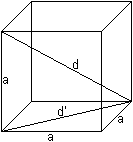... There are 12 equal edges.  Three edges meet in a corner and stand perpendicularly on each other in pairs.  The length of an edge is a. There are There are 12 square diagonals of the length d' = sqr(2)a.  There are four space diagonals of the length d = sqr(3)a.  The volume is V=a³, the surface ist O = 6a².  The circumscribed sphere has the radius R = (1/2)sqr(3)a, the inscribed sphere the radius r = (1/2)a. The radius of the midsphere is rk = (1/2)sqrt(2)a.

Views of a Cube top
Cubes in Perspective
 ...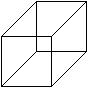... If students shall draw the picture of a cube in perspective and you tell them before that all edges of the cube are equal some of them draw the picture on the left.  You don't think that this is a good view of a cube. It is more a column. Obviously the sloping lines must be shortened.
There is the question, how much you must shorten to get a nice view. If you have the choice of  the following four drawings most people would choose picture 3 as the best.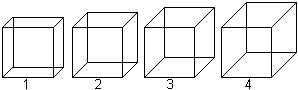The sloping line is about half as large as the true length of an egde. Thus you get the ratio k=1/2.

The ratio depends on the angles of the sloping lines. The three following statements produce good pictures:The mathematical base is the "sloping parallel projection", where all ratios and angles are possible. You choose simple angles and simple ratios (Book S).

There is Pohlke's theorem (also called "Main theorem of the axonometry"):
Every real 2d-tripod OABC can be produced by parallel projection of a Cartesian 3d-tripod O'A'B'C'.
(http://www.math-inf.uni-greifswald.de/mathematik+kunst/themen.html)

More Cubes in Perspective
 ...... Actually the drawing with equal edges on the left was not used as a picture of a cube. Nevertheless you may take it. The advantage is that the length in direction of the lines are real. The "isometric perspective" (30° instead of 45°) is not so distorted and preferred.

Central Projection
 ...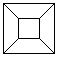... Think of the edge model of a cube.  If you project it with light which comes from a point you can get the picture on the left.

Nets of a Cube
 ...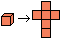... Think of a paper cube and cut it along the edges. You get nets of a cube. There are 11 nets. ......

Stereogram
You can see the cube three-dimensionally  in the following picture.More on my web page Stereogram

Shadow pictures of a rotating cube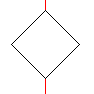For several years the "Deutsche Museum" in Munich has the department "Das Mathematische Kabinett". A nice exhibit is a rotating edge model of a cube. It is produced by parallel rays. You see the figures on the left one after the other on a screen (square on the top, hexagon with diagonal and a rectangle with a middle line). You don't believe that these figures come from a cube and must look twice. The cube is positioned in the way, that two edges in opposite lie on top of each other. An axis (red) is interrupted in the middle.

Building a Cube top
Model with squares
 ...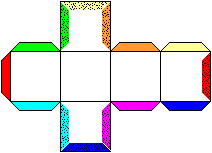... This is the well known way to build a cube.  Draw a net and give the edges stripes for gluing.  Flex the stripes and glue them on the pointed fields with the same colour. The cube will be closed with a lid on the right.

Model with edges
There are different methods of building cubes with rods.

>Remove the top of a match and connect the sticks with two-component gluing.

>Take a toothpick or chopstick and connect them with balls of modelling clay.

>Cut equal wire pieces with pincers and connect them by soldering, so that they form a cube. It is clever to fix the three pieces of a corner and let them touch before soldering. You can manage if  you use "die helfende Hand" (the helping hand) for two ends and the real hand for the third end.

>Cut drinking straws in equal pieces and connect them with tripods of florist's wire or paper clips.

More exact: There are building kits, which use 12 straws and 8 tripods of plastic (One red tripod is removed below in the photo).
You can make tripods (below, dark green) yourself. You bend them twice in six lines. There are bends in the tops, the two ends of a wire meet in the middle....... You can find a clever fashionable toy:  Bar magnets form the edges, steel balls the corners.  You pay 14€  for building kits of a cube (Springtime 2002).  In the meantime (Autumn 2003) there are different firms, which produce them. Competition is good for the price.

Symmetries of a Cube  top
The centre of the cube is a symmetry centre.

 ...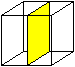... The cube has nine symmetry planes.  Three planes lie parallel to the side squares and go through the centre (picture).  Six planes go through opposite edges and two body diagonals. They divide the cube into prisms.

You can find 13 rotation axes. If you turn around one of these axes, the cube goes back to itself.
The following picture illustrates these facts. The numbers under the cubes indicate the number of turns.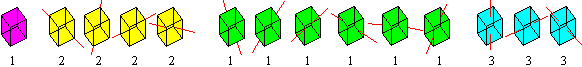Interior Views of a Cube top
All diagonals of the surface squares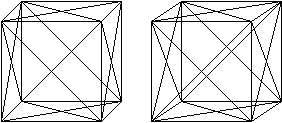All spatial diagonals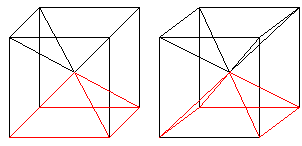Cube with cut corners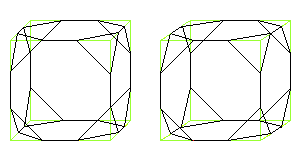Cut the corners of a cube. Divide the edges in three equal pieces to do this.
You get a body formed by 6 octogons and 8 equilateral triangles.

Cuboctahedron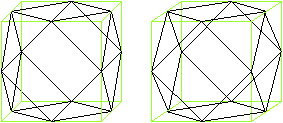Cut the corners of a cube. Take half the edges.
You get a body formed by 6 squares and 8 equilateral triangles. .

Tetrahedron in the cubeDraw some square diagonals and you get a tetrahedron.

Octahedron in the cube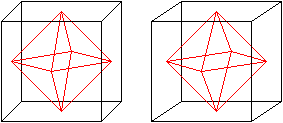Join the centres of the squares by lines. You get an octahedron.
More: If you join the centres of the triangles of a octahedron, a cube develops again.
Cube and octahedron are dual to each other.

Three pyramids of equal volumes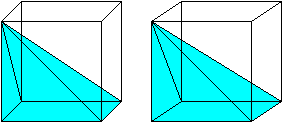The largest square inside a cube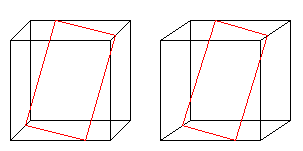The red square is the largest square which fits a cube.
The corners of the square divide the edges in 1:3 (Book G).

Cube in the cube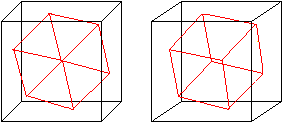The red cube is the smallest cube which touches all sides of the black cube (more on web page E).

Hexagon inside a cube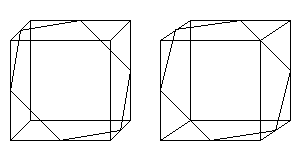Join centres of some edges. You get an intersection through the cube.
The intersection line is a hexagon of the edge length sqrt(2)/2*a, if a is the edge of the cube.

A spatial equilateral hexagon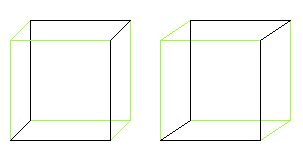Delos Problem (problem of cube duplication) top
The ancient Greeks could get rid of the plague after an answer of the oracle of Delos, if they doubled the volume of its cube altar. (This is one version of the legend.)
 ...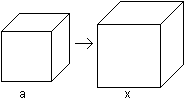... The problem of the cube duplication goes to the equation 2a³=x³  and the solution x=a*2^(1/3).  This was no solution to the old Greeks, because the distance x had to be found from the length  only with circle and ruler . Now we know that this problem is unsolvable, because only terms with square roots are possible to be constructed. Circles and straight lines lead to linear and quadratic equations, which x³=a³ does not belong  to.
Two other problems also are unsolvable for the same reason: The conversion of the circle into a square with the equal area ("squaring the circle") and the division of any angle in three equal parts ("three-division of an angle").

Polycubes   top
You can form polycubes, if you add cubes touching in one or several squares.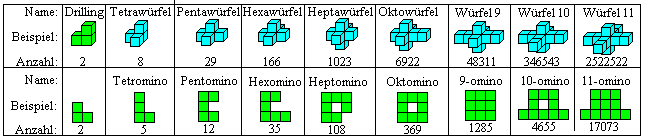Die Zahlen geben die Anzahl der jeweiligen Würfelkörper einer Sorte an. (Webseite K)

There are many puzzles based on polycubes. Here is my "hit list":I describe the puzzles on other places of my homepage. There are 1 Soma cube, 2 Mac Mahons Coloured Cubes, 3 Rubik's cube, 4 Happy Cube, and 5 Origami cube
More cube pages are tetracubes, snake cube, magic cube, classic puzzle cube, (and Dice).

Hypercube (4dimensional cube) topYou find a description on my web page hypercube.

Optical Illusions top
If you like, every representation of a cube in the plane is a optical illusions. You think you see a 3D cube, though the drawing plane is 2D.

Well known illusions with cubes are "tilt figures". I restrict on them.
 ...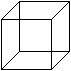... The Necker cube (on the left) shows a cube in two perspectives (on the right).  You switch over from one sight to the other. A square is sometimes in front or in the back. You can only see one view at one moment. ...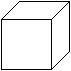...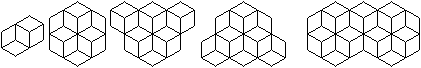You have the questions:
Right or left?  From below or above?   Three or five cubes? Five or three cubes? How many cubes?

The five pictures above are ambiguous. You see the cube from below or above.Do you look on a tower or into a hole?

The Cube as a Building   top
A building in the shape of a cube is not practical. Maybe because of that architects and artists have taken the challenge and became creative. The results are many original show-pieces and sculptures all over the world.
Here is a small look back on the unforgettable Expo 2000 in Hanover, Germany.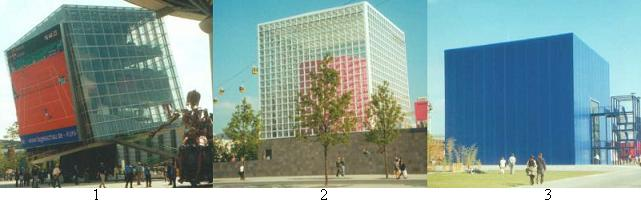1 The T-Digit of the "Deutsche Telekom" was a centre of the exhibition area. You could look at a large screen sitting on the steps of show stairs where (even in the sunshine) an impressive light TV picture was to be seen. Have a look at the French Open in Paris.

2 The exhibition cube of Mexico was seen from nearly every point of the area.

3 The Iceland cube was unforgettable: It was dark blue and over it there was a water layer. Inside there was a round water pond whose surface was used as a screen for a movie about Iceland in the bird's-eye view. In the end of the performance an artificial geyser erupted.

4 I forgot to take a photo of the Norwegian cube building equipped with a waterfall :-(.
 ...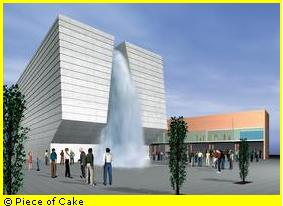... Therefore I restrict on a model, which you could find on the internet before the Expo opening. (The waterfall didn't become so mighty.)  You entered the "room of silence" after a slight shower. You could sit on the hard floor for some minutes and watch the Norwegian sceneries on all six squares. From time to time stones rolled down the rocks (only acoustically). It was not allowed to talk. My wife... (censorship!)

The Cube on the Internet   top

German

H. B. Meyer (Polyeder aus Flechtstreifen)
Hexaeder, Würfel (spezial)

Richard Mischak
Würfel

Wikipedia
Würfel (Geometrie)Platonischer KörperDelisches ProblemExpo 2000

English

David Eppstein (Geometry Junkyard)
Box in a Box

Eric Weisstein (MathWorld)
Cube, Prince Rupert's Cube

H. B. Meyer (Polyeder aus Flechtstreifen)
Cube, Cube (special)

Wikipedia
Cube, Platonic solid, Doubling the cube, Expo 2000

References  top
(G) Martin Gardner: Mathematischer Karneval, Ullstein Berlin 1977 (Seite 61)
(S) Hans Simon, Kurt Stahl: Mathematik, Formeln und Gesetze, Leipzig 1979 (Seite 471ff.)
(K) Michael Keller (früher: http://members.aol.com/wgreview/polyenum.html)
(E) David Eppstein (http://www.ics.uci.edu/~eppstein/junkyard/box-in-box.html)

Feedback: Email address on my main page